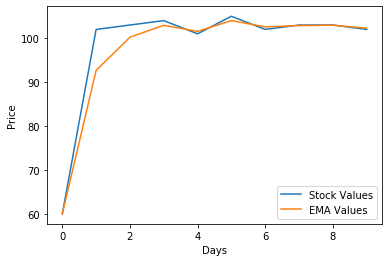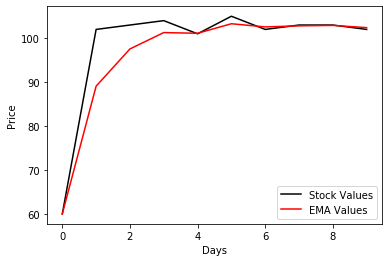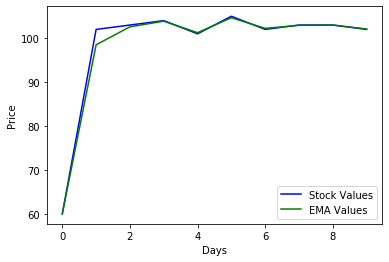GeeksforGeeks App
Open AppBrowser
Continue

# How to Calculate an Exponential Moving Average in Python?

Moving Averages are financial indicators which are used to analyze stock values over a long period of time. i.e. Average value for that long period is calculated. Exponential Moving Averages (EMA) is a type of Moving Averages. It helps users to filter noise and produce a smooth curve. In Moving Averages 2 are very popular.

1. Simple Moving Average
2. Exponential Moving Average

Simple Moving Average just calculates the average value by performing a mean operation on given data but it changes from interval to interval. But whereas in Exponential Moving Average also uses Simple Mean Average in calculating its average but gives more weightage to the newly added value as the latest value has more weightage.

Formula

EMAToday=( ValueToday*(Constant/ (1+No. Of Days)) )+( EMAYesterday*(1-(Constant/(1+No. Of Days))) )

Exponential Moving Average value for Today is calculated using Previous Value of Exponential Moving Average. Here the older values get less weightage and newer values get more weightage. This decrease in weightage of values is calculated using Constant value called Decay.  So as the number of days gets increases value becomes less significant. It helps to prevent the fluctuations of values.

## Using ewm method in Pandas

The exponential Weighted Mean method is used to calculate EMA which takes a decay constant as a parameter.

Syntax

DataFrameName.ewm(com=value)

Example 1:

As the plot of EMA values is little smoothened when compared to Original Stock values indicates the nature of Exponential Moving Averages.

## Python3

 `# import necessary packages``import` `pandas as pd``import` `matplotlib.pyplot as plt` `# create a dataframe``stockValues ``=` `pd.DataFrame(``    ``{``'Stock_Values'``: [``60``, ``102``, ``103``, ``104``, ``101``,``                      ``105``, ``102``, ``103``, ``103``, ``102``]})` `# finding EMA``# use any constant value that results in``# good smoothened curve``ema ``=` `stockValues.ewm(com``=``0.4``).mean()` `# Comparison plot b/w stock values & EMA``plt.plot(stockValues, label``=``"Stock Values"``)``plt.plot(ema, label``=``"EMA Values"``)``plt.xlabel(``"Days"``)``plt.ylabel(``"Price"``)``plt.legend()``plt.show()`

OutputExample 2:

In the below code we will take the same DataFrame we used above with a different com value which is a higher value compared to that of above. It will be passed as an argument to ewm method.

## Python3

 `# import necessary packages``import` `pandas as pd``import` `matplotlib.pyplot as plt` `# create a dataframe``stockValues ``=` `pd.DataFrame(``    ``{``'Stock_Values'``: [``60``, ``102``, ``103``, ``104``, ``101``, ``105``,``                      ``102``, ``103``, ``103``, ``102``]})` `# finding EMA``# used constant value as 0.8``ema ``=` `stockValues.ewm(com``=``0.8``).mean()` `# Comparison plot b/w stock values & EMA``plt.plot(stockValues, label``=``"Stock Values"``, color``=``"black"``)``plt.plot(ema, label``=``"EMA Values"``, color``=``"red"``)``plt.xlabel(``"Days"``)``plt.ylabel(``"Price"``)``plt.legend()``plt.show()`

OutputExample 3:

Here we will consider the same DataFrame we used in the above 2 examples with a different com value which is almost close to zero, passed as an argument to ewm method.

## Python3

 `# import necessary packages``import` `pandas as pd``import` `matplotlib.pyplot as plt` `# create a dataframe``stockValues ``=` `pd.DataFrame(``    ``{``'Stock_Values'``: [``60``, ``102``, ``103``, ``104``, ``101``, ``105``,``                      ``102``, ``103``, ``103``, ``102``]})` `# finding EMA``# com value=0.1 (0 approx)``ema ``=` `stockValues.ewm(com``=``0.1``).mean()` `# Comparison plot b/w stock values & EMA``plt.plot(stockValues, label``=``"Stock Values"``, color``=``"blue"``)``plt.plot(ema, label``=``"EMA Values"``, color``=``"green"``)``plt.xlabel(``"Days"``)``plt.ylabel(``"Price"``)``plt.legend()``plt.show()`

OutputMy Personal Notes arrow_drop_up